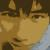## 思维被Lisp的语法所绑架

pollex 发布于 2014/05/22 14:21

```int f(int x, int y, int z)
{
return x * y + (x*2 + y*2 + z);
}```

```(define (f x y z)
(+ (* x y)
(+ (+ (* x 2) (* y 2)) z)))```

```class app
{
public static void main(String args[])
{
System.out.println("hello world!");
}
}```

```#include <stdio.h>

int main(int argc, char* argv[])
{
if (argc < 2)
{
printf("argc < 2\n");
return 1;
}
printf("arg 1 = %s\n", argv);
return 0;
}```

```data SEGMENT
msg DB 'Hello, world.\$'
data ENDS
code SEGMENT
ASSUME CS:code,DS:data
start:
MOV AX,data
MOV DS,AX
LEA DX,msg
MOV AH,9h
INT 21h
MOV AX,4C00h
INT 21h
code ENDS
END start```

S表达式从来就不优美，不是样子不优美，而是思维不优美，甚至很丑。机器有机器的方式，人类有人类的方式，就算是汇编这种机器语言，也要弄出很多人类能理解的助记符，因为写程序的是人类而不是机器。所以才需要语法这种东西，如果不是为了让人能理解，那么今天的计算机还要那么多东西干嘛？直接让人来二进制读写不就好了？S表达式正是这个观念，把机器应该做的事变成人必须做的事。

0